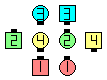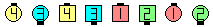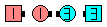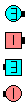Definitions of Square Dance Calls and Concepts

Double Pass Thru \$B\$^\$?\$O(B R-H 1/4 Tag \$B\$+\$i(B.

Centers \$B\$O(B Pass Thru \$B\$r\$7\$F(B, Right | Left Turn To A Line and Spread \$B\$r\$7(B Tidal Line \$B\$N(B #1 (Very End) \$B\$H(B #3 \$B\$H\$J\$j(B, \$BB>\$N?M\$O(B Step Ahead and Veer Right | Left \$B\$r\$7(B Tidal Line \$B\$N(B #2 \$B\$H(B #4 \$B\$K\$J\$j\$^\$9(B.Strut Right\$B\$NA0(B \$B8e(B

\$BCm
• \$B\$b\$7(B 2 \$B\$D\$N(B direction \$BM?\$(\$i\$l\$?\$H\$-\$O(B, \$B%*%j%8%J%k\$N(B Centers \$B\$,(B 1 \$B\$DL\\$N(B direction \$B\$r(B, \$B%*%j%8%J%k\$N(B Outsides \$B\$O(B 2 \$B\$DL\\$N(B direction \$B\$r;H\$\$\$^\$9(B. \$B\$b\$7(B 1 \$B\$D\$@\$1\$N(B direction \$B\$,M?\$(\$i\$l\$?\$H\$-\$O(B, \$BA40w\$G\$=\$N(B direction \$B\$r;H\$\$\$^\$9(B.
• Centers \$B\$O(B, \$B%3!<%k\$,(B Strut Left \$B\$G\$"\$C\$F\$b(B, \$B>o\$K(B\$B1&(B\$B8*\$GDL\$j\$^\$9(B. \$B\$b\$7(B, \$B:8(B\$B8*\$GDL\$j\$?\$\$\$J\$i(B, \$B%3!<%i!<\$O(B Left Strut Right|Left \$B\$H8@\$o\$J\$1\$l\$P\$J\$j\$^\$;\$s(B.
• \$B\$b\$7(B, \$B\$"\$J\$?\$XM?\$(\$i\$l\$?(B direction \$B\$,(B Right \$B\$N\$H\$-\$O(B, Beau \$B\$H\$J\$k(B (\$B\$9\$J\$o\$A(B, \$B<+J,\$N0LCV\$O(B Right-hand Tidal Wave \$B\$K\$\$\$k\$h\$&\$K(B) \$B\$O\$:\$G\$9(B. \$BF1MM\$K(B, \$BM?\$(\$i\$l\$?(B direction \$B\$,(B Left \$B\$N\$H\$-\$O(B, Belle \$B\$G\$H\$J\$k\$O\$:\$G\$9(B.
• \$B%R%s%H(B: \$B%*%j%8%J%k\$N(B Outsides \$B\$O(B, Centers \$B\$,%3!<%k\$r=*\$(\$kD>A0\$^\$G(B, \$BF0\$-;O\$a\$k\$Y\$-\$G\$O\$"\$j\$^\$;\$s(B. \$B\$3\$N\$3\$H\$G(B, Outsides \$B\$,F0\$/\$?\$a\$N==J,\$J>l=j\$,F@\$i\$l\$^\$9(B.

Single Strut Right | Left (And Right | Left) [C3B] (Lee Kopman 1977): Single Double Pass Thru, Single R-H 1/4 Tag, \$B\$^\$?\$OB>\$NE,@Z\$J(B formation \$B\$+\$i(B. Centers \$B\$O(B Pass Thru \$B\$r\$7\$F(B, Single Right|Left Turn To A Line and Spread \$B\$r\$7(B, \$BB>\$N?M\$O(B Step Ahead and Single Veer Right|Left \$B\$r\$7\$^\$9(B. \$BIaDL(B Wave \$B\$^\$?\$O(B Line \$B\$G=*\$o\$j\$^\$9(B.Single Strut Right And Left\$B\$NA0(B \$B8e(B